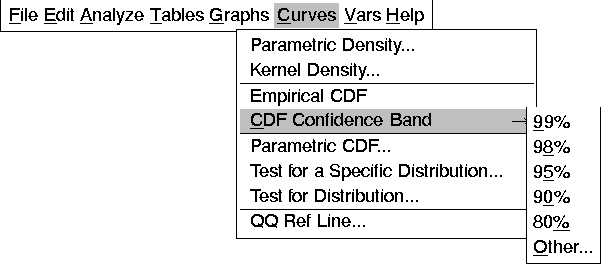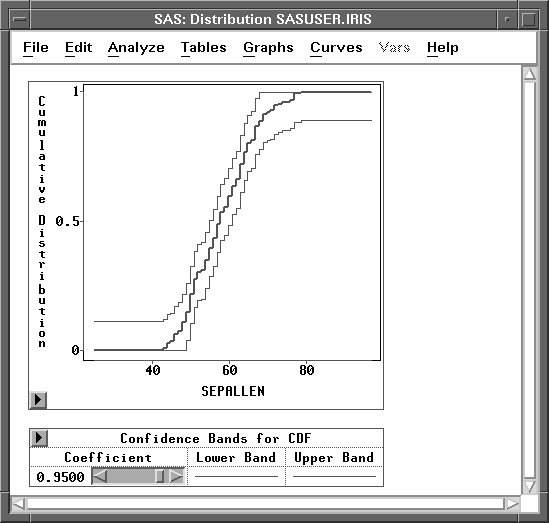Distribution Analyses

## CDF Confidence Band

The confidence band gives a confidence region for the population distribution. The critical values given by Feller (1948) for the completely specified hypothesized distribution are used to generate the confidence band. All parameters in the hypothesized distribution are known. The null hypothesis that the population distribution is equal to a given completely specified distribution is rejected if the hypothesized distribution falls outside the confidence band at any point.

You specify the confidence coefficient in the cumulative distribution options dialog or by choosing Curves:CDF Confidence Band.Figure 38.27: CDF Confidence Band Menu

Figure 38.28 displays an empirical distribution function and a 95% confidence band for the cumulative distribution function. Use the Coefficient slider to change the coefficient for the confidence band.Figure 38.28: CDF Confidence Band